﻿ 一种复杂环境下的电力线检测方法
«上一篇文章快速检索 高级检索

 应用科技2019, Vol. 46Issue (5): 51-56  DOI: 10.11991/yykj.2019010090

### 引用本文ZHANG Qiuyan, YANG Zhong, CHEN Cong, et al. A power line detection method in a complex background[J]. Applied Science and Technology, 2019, 46(5): 51-56. DOI: 10.11991/yykj.201901009.### 文章历史

1. 贵州电网有限责任公司 电力科学研究院，贵州 贵阳 550002;
2. 南京航空航天大学 自动化学院，江苏 南京 211106

A power line detection method in a complex background
ZHANG Qiuyan 1 , YANG Zhong 2, CHEN Cong 2 , HU Guoxiong 2 , HAN Jiaming 2 , XU Changliang 2 , LAI Shangxiang 2
1. Electric Power Research Institute of Guizhou Power Grid Co., Ltd., Guiyang 550000, China;
2. College of Automation Engineering, Nanjing University of Aeronautics and Astronautics, Nanjing 210016, China
Abstract: Fast and accurate detection of power lines from images taken by UAV with a complex background is a key problem in the work of tree block cleaning robots. In this paper, by analyzing the characteristics of power lines in images, a method for power line detection in a complex background is proposed. First, the equalization processing is performed on original images to increase the contrast between power lines and background. Then the Hessian algorithm is used to detect the edge of power lines, further the Hough transform is used to extract power line and linear screening method based on k-means++ cluster is used to extract the power line. The experimental results show that the method has higher accuracy and completion of power line extraction in a complex background.
Keywords: complex background    power line detection    image enhancement    Hessian algorithm    Hessian algorithm    Hough transform    cluster

1 图像预处理

 $\begin{array}{l} F_{\rm{Gray}}(x,y) = 0.299 \times R(x,y) + 0.578 \times G(x,y) + \\ \;\;\;\;\;\;\;\;\;\;\;\;\;\;\;\;\;\;\;\;\;\;0.114 \times B(x,y) \\ \end{array}$ (1)

 $P(r) = \frac{{{n_r}}}{N},0 \leqslant r \leqslant 1$

 $s = T(r) = \sum\limits_{j = 1}^k {\frac{{{n_j}}}{N} = } \sum\limits_{j = 1}^k {P({r_j})} ,k = 0,1, \cdots ,255$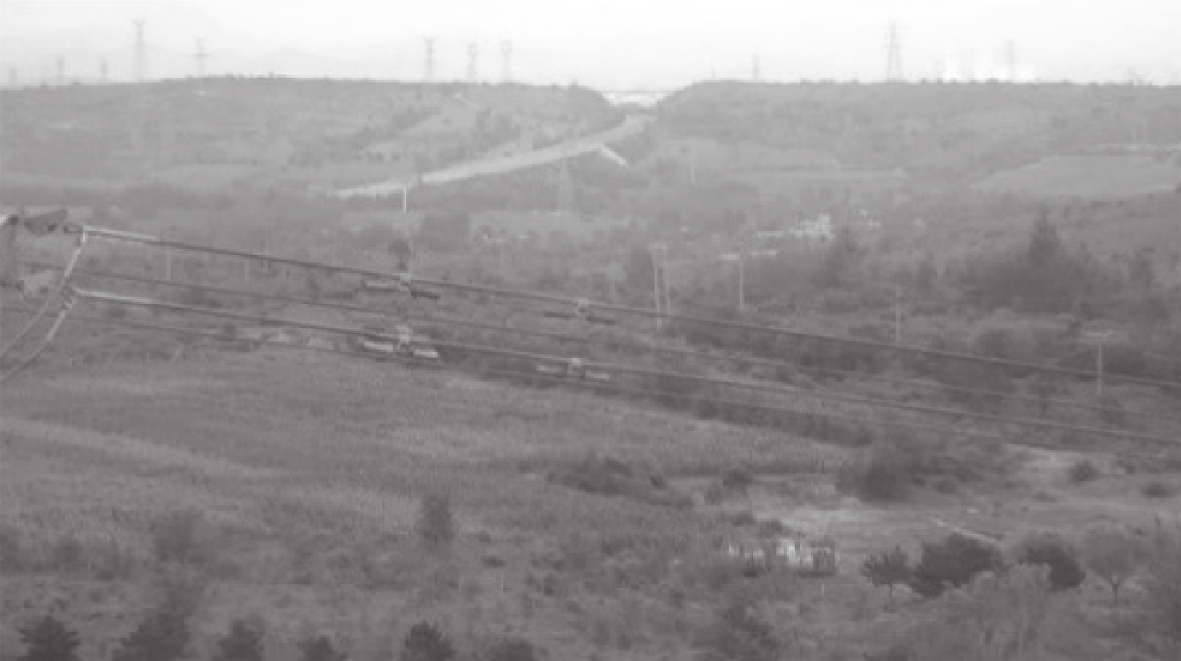Download: 图 1 原灰度图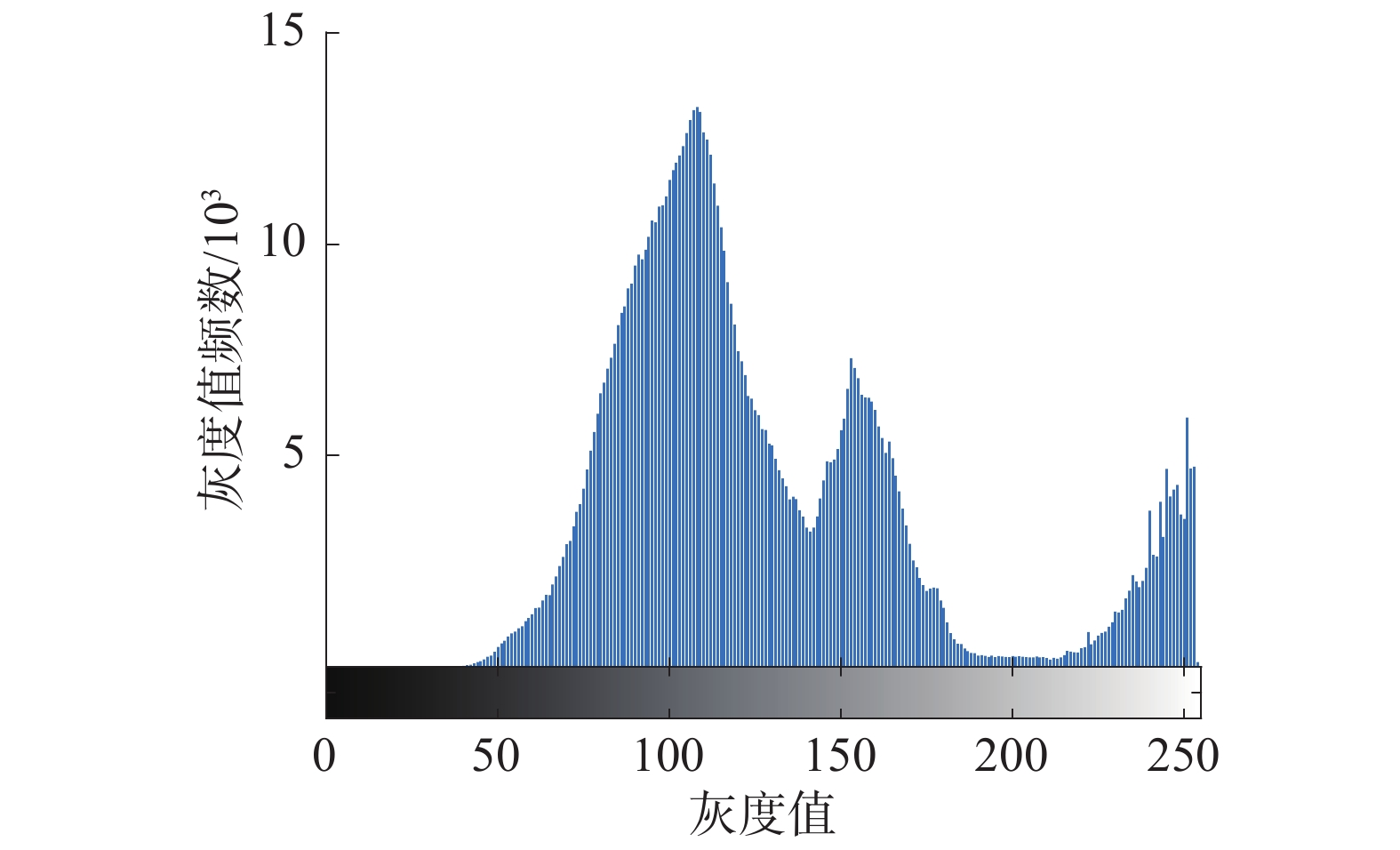Download: 图 2 原灰度直方图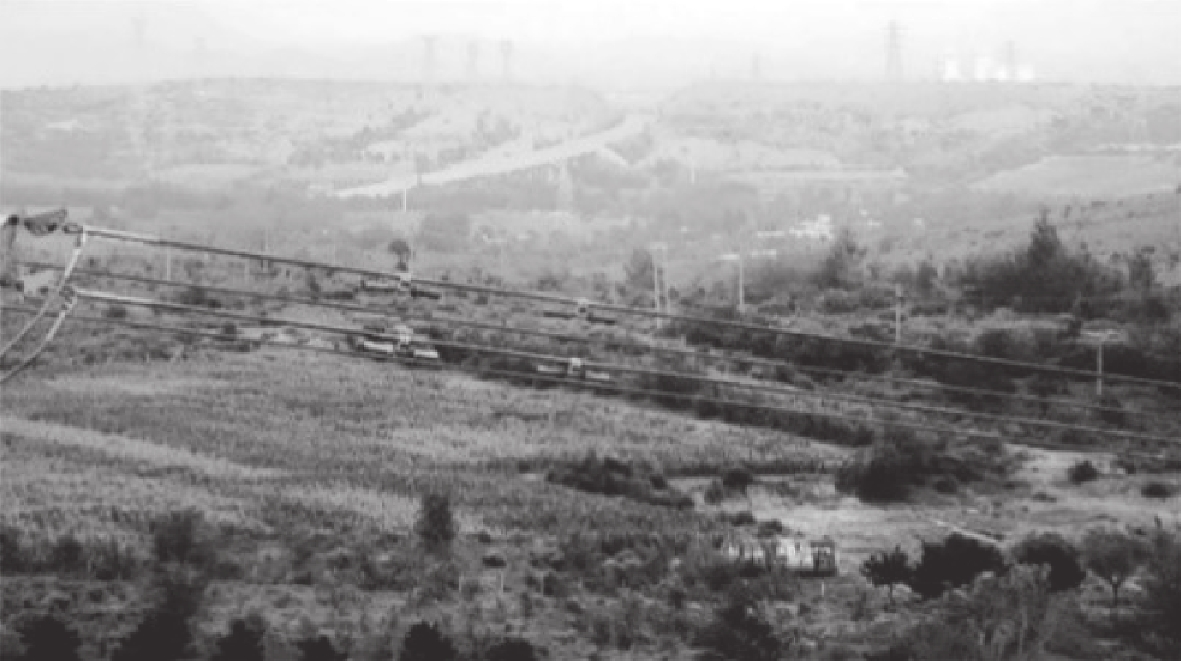Download: 图 3 直方图均衡化处理后的灰度图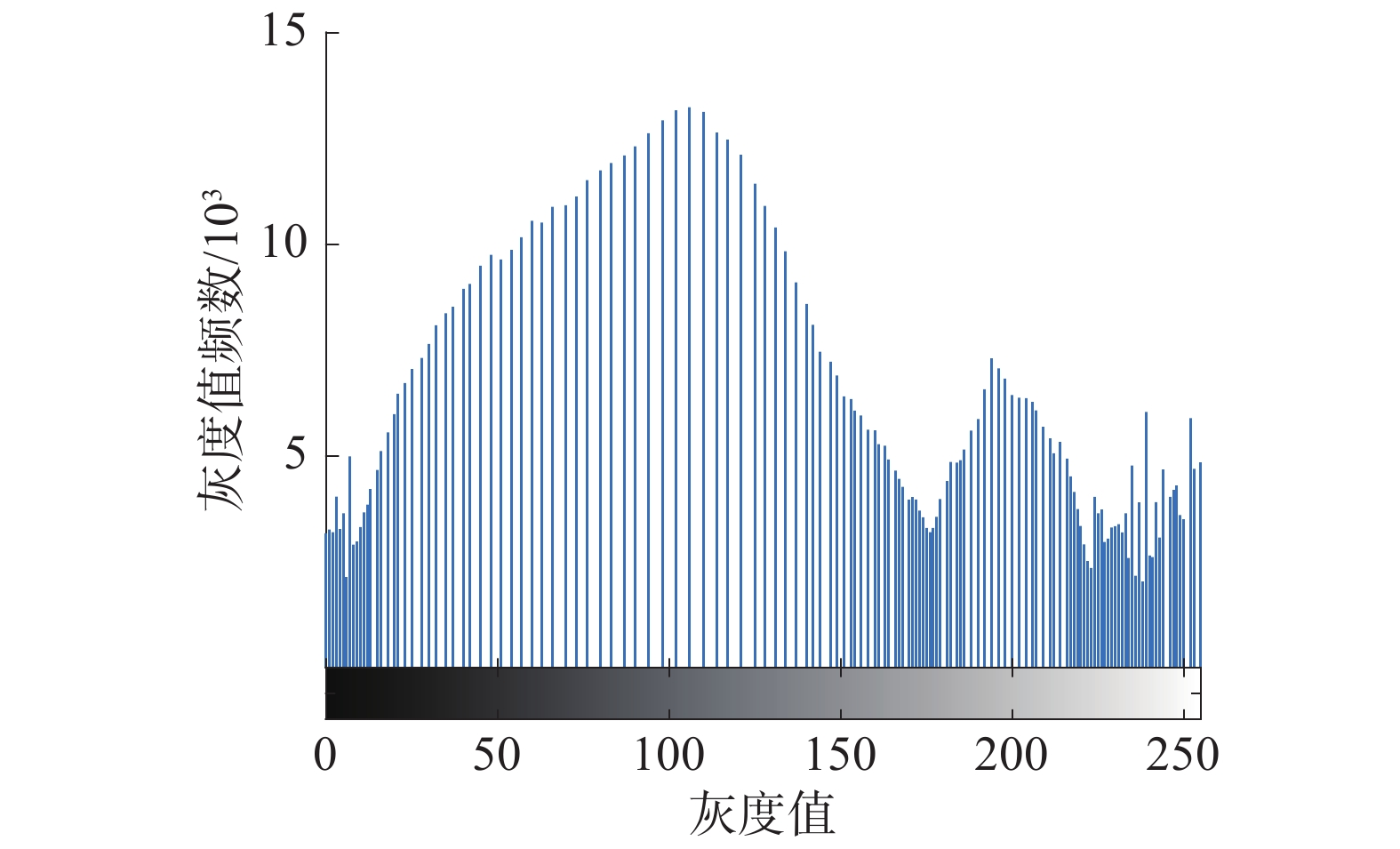Download: 图 4 直方图均衡化处理后的灰度直方图
2 电力线边缘检测

Hessian矩阵是一个多元函数的二阶偏导数构成的方阵，描述了函数的局部曲率。在图像处理中，Hessian矩阵可以进行边缘与特征点检测、消除边缘响应，眼底图像血管增强与分割中基于Hessian矩阵的Frangi滤波算法，即利用了Hessian矩阵对眼底血管边缘进行了增强

 $\left\{ \begin{gathered} {G_{xx}} = \frac{{{\partial ^2}{G_\sigma }(x,y)}}{{\partial {x^2}}} \\ {G_{xy}} = \frac{{{\partial ^2}{G_\sigma }(x,y)}}{{\partial x\partial y}} \\ {G_{yy}} = \frac{{{\partial ^2}{G_\sigma }(x,y)}}{{\partial {y^2}}} \\ \end{gathered} \right.$

 $\left\{ \begin{gathered} {I_{xx}} = {G_{xx}} * \operatorname{Im} g \\ {I_{xy}} = {G_{xy}} * \operatorname{Im} g \\ {I_{yy}} = {G_{yy}} * \operatorname{Im} g \\ \end{gathered} \right.$
 ${{H}} = \left( {\begin{array}{*{20}{c}} {{I_{xx}}}&{{I_{xy}}} \\ {{I_{xy}}}&{{I_{yy}}} \end{array}} \right)$Download: 图 5 Sobel算法检测出的边缘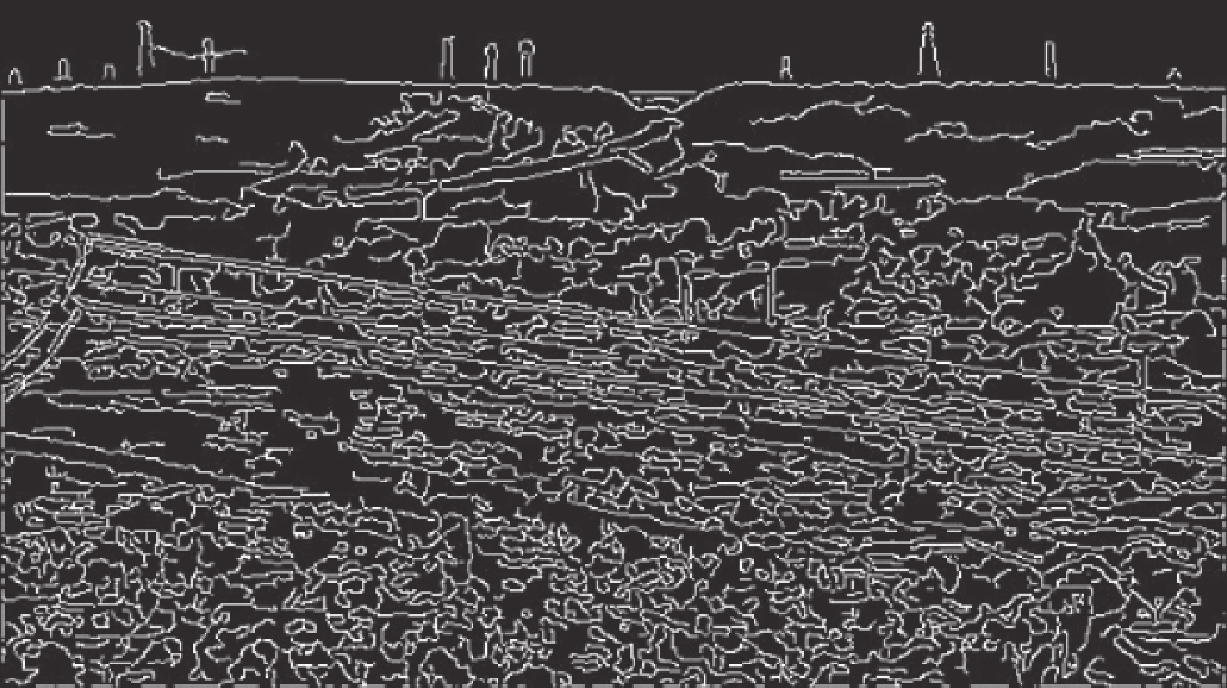Download: 图 6 Canny算法检测出边缘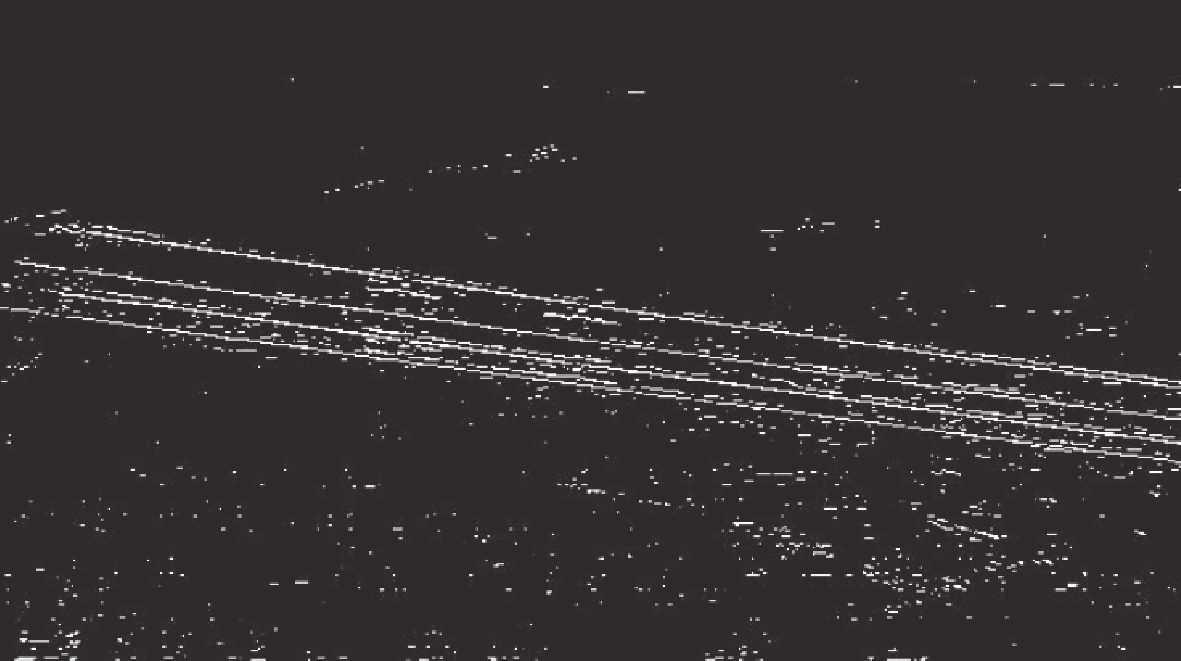Download: 图 7 Hessian算法检测出的边缘
3 电力线提取 3.1 Hough变换

 $y = kx + b$

 $d = {x_0}\cos \theta + {y_0}\sin \theta$

3.2 直线筛选方法

k−means算法是一种广泛应用的基于划分的聚类算法，以距离作为数据实例间相似性度量的标准，数据实例之间的距离越小，相似性即越高，则属于同一个类簇的可能性越大。但k−means算法开始时对k个初始聚类中心的选择会对聚类结果有较大的影响，可能无法得到有效的聚类结果。k−means++算法获得的初始聚类中心之间的相互距离尽可能远，可以有效地避免这种情况出现。

k−means++算法的步骤如下：

1）从输入的数据集 $X$ 中随机选择一个点，将其作为初始的聚类中心；计算数据集 $X$ 中的每一个点 $x$ 与已选择的最近聚类中心的距离 $D(x)$ ；计算样本点 $x$ 被选为下一个聚类中心的概率 $P$

 $P = \frac{{D{{(x)}^2}}}{{\displaystyle\sum\nolimits_{x \in X} {D{{(x)}^2}} }}$

2）选择概率 $P$ 值大的样本点 $x$ 作为新的聚类中心，直到选出所需的k个聚类中心。再利用选出的k个初始的聚类中心进行k−means算法。

4 实验结果与分析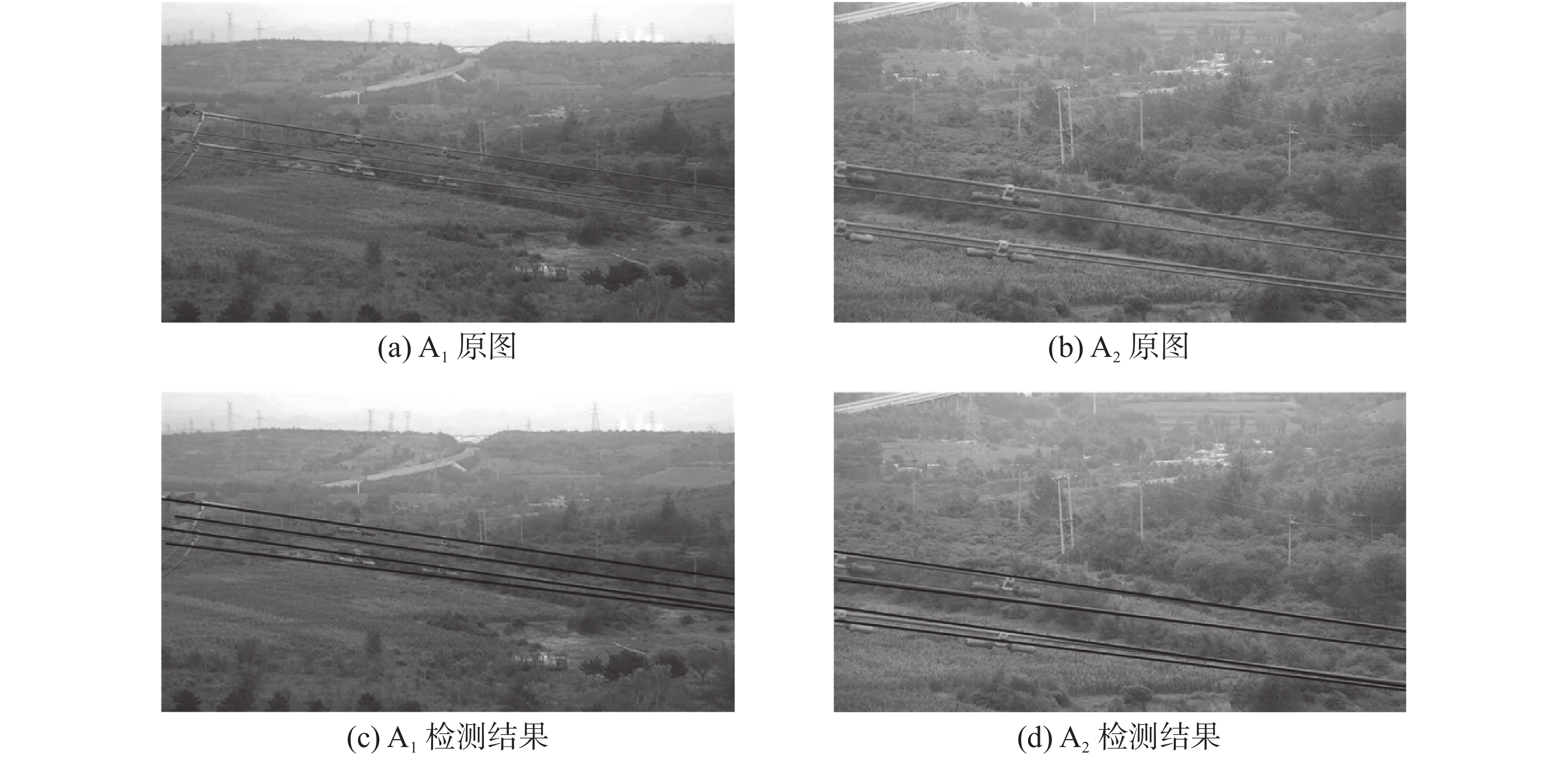Download: 图 8 场景A电力线检测结果Download: 图 9 场景B电力线检测结果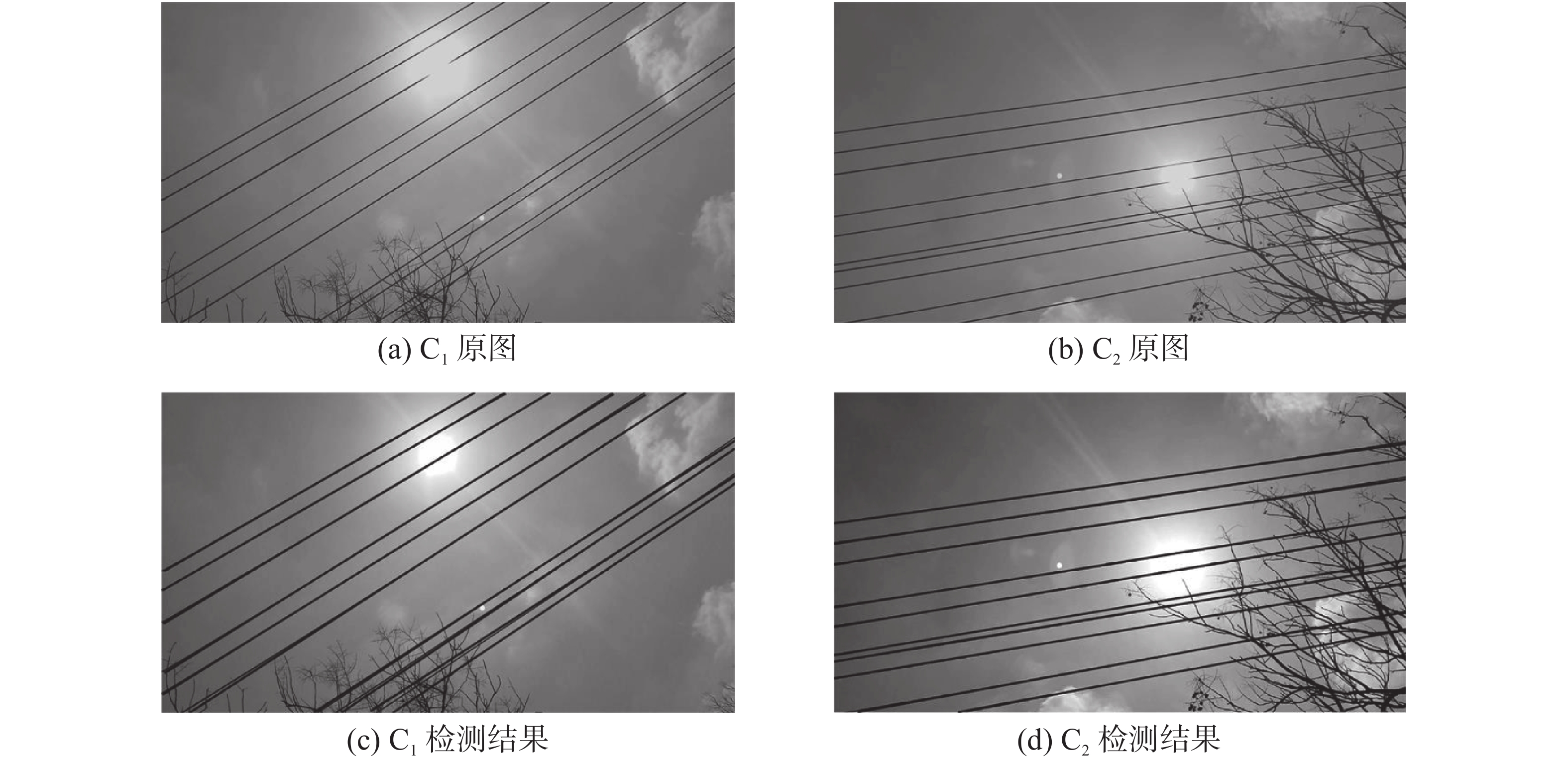Download: 图 10 场景C电力线检测结果表 1 电力线提取结果表 2 斜率聚类结果

5 结论

  胡彬, 周宗国, 杨时宽, 等. 输电线路走廊树障清理相关技术分析[J]. 民营科技, 2018(9): 126-127. (0)  蒋超, 牛宏侠. 基于改进Radon变换的直线钢轨识别算法[J]. 铁道标准设计, 2017, 61(4): 19-22. (0)  李欣, 刘美秀, 刘迪. 复杂背景下高层建筑钢结构板孔心影像坐标量测[J]. 武汉大学学报(工学版), 2016, 49(1): 143-147. (0)  赵乐, 王先培, 代荡荡, 等. 复杂背景下电力线自动提取算法[J]. 高电压技术, 2019, 45(1): 218-227. (0)  GROMPONE VON GIOI R, JAKUBOWICZ J, MOREL J M, et al. LSD: a fast line segment detector with a false detection control[J]. IEEE transactions on pattern analysis and machine intelligence, 2010, 32(4): 722-732. DOI:10.1109/TPAMI.2008.300 (0)  张从新, 赵乐, 王先培. 复杂地物背景下电力线的快速提取算法[J]. 武汉大学学报(工学版), 2018, 51(8): 732-739. (0)  FRANGI A F, NIESSEN W J, VINCKEN K L, et al. Multiscale vessel enhancement filtering[C]//Proceedings of the 1st International Conference on Medical Image Computing and Computer-Assisted Intervention. Cambridge, MA, USA: Springer, 1998: 130−137. (0)  孙实超. 无人机航拍图像的输电线识别方法[D]. 南宁: 广西大学, 2016. (0)  吴夙慧, 成颖, 郑彦宁, 等. k-means算法研究综述[J]. 现代图书情报技术, 2011(5): 28-35. (0)  ARTHUR D, VASSILVITSKII S. k-means++: the advantages of careful seeding[C]//Proceedings of the Eighteenth Annual ACM-SIAM Symposium on Discrete Algorithms. New Orleans, Louisiana, USA: ACM, 2007: 1027−1035. (0)